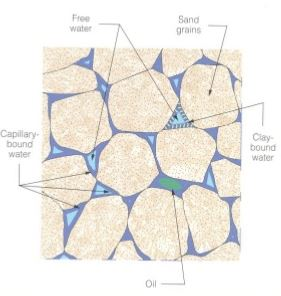# Fluid Saturation

Fluid saturation is how much each fluid is present in pore spaces of a rock. This will affect the ability of each fluid flow through porous media. This is one of critical values for reservoir engineering since many engineering calculations need fluid saturation values.Fluid Saturation in Rock

Fluid saturation is expressed in terms of volume of fluid divided by total pore space.

Gas saturation (Sg) = Vg ÷ Vp

Oil saturation (So) = Vo ÷ Vp

Water saturation (Sw) = Vw ÷ Vp

Total saturation of fluid is equation to 1, therefore this can mathematically be expressed like this.

Sg + So + Sw = 1

Where;

Vg = volume of gas in pore space

Vo = volume of oil in pore space

Vw = volume of water in pore space

## How To Measure Fluid Saturation

Two methods to determine fluid saturation are 1) core sample and 2) wireline logging. Core sample is a direct method because it is a physical measurement. However, wireline logging is an indirect method because the values are derived from mathematical models.

## Fluid Saturation Video by SPE

References

Abhijit Y. Dandekar, 2013. Petroleum Reservoir Rock and Fluid Properties, Second Edition. 2 Edition. CRC Press.

L.P. Dake, 1983. Fundamentals of Reservoir Engineering, Volume 8 (Developments in Petroleum Science). New impression Edition. Elsevier Science.

Tarek Ahmed PhD PE, 2011. Advanced Reservoir Management and Engineering, Second Edition. 2 Edition. Gulf Professional Publishing.

Share the joy